# Custom report by hour

I want make a report from begin work period to end time, hour by hour show the payment.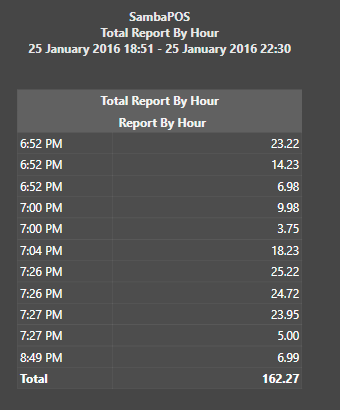For example: i want the report like
Time | payment amount
6-7 | 43.45
7-8 | 110.79
8-9 | 6.99
Total | 162.27
so anyone can tell me how to do it will like this?Thanks a lot.

Report Template:

``````[Sales Per Hour: 15, 25, 15, 20, 20]
>Hour|Sales|Tx|AvgTx|%Sales
@@SalesPerHour:6,22
``````

Change the `6,22` parameter above to set your Start and End hours. For example, a full day would be:

``````@@SalesPerHour:0,23
``````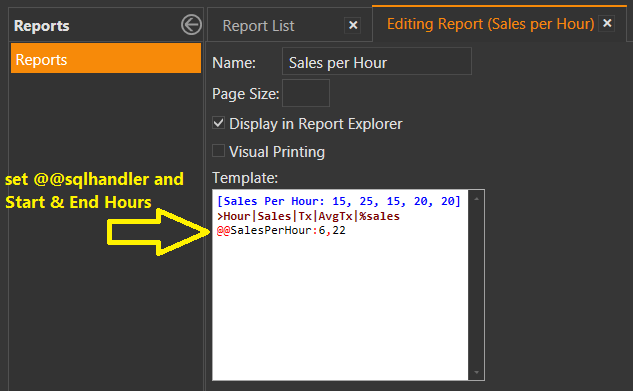`@@SalesPerHour` (SQL Script in `Automation > Scripts`)

``````declare @StartDate datetime = convert(varchar(25),'{Start}',126)
declare @EndDate datetime = convert(varchar(25),'{End}',126)
declare @StartHour int = @1 -- set the First Hour you want to track
declare @EndHour int = @2  -- set the Last Hour you want to track

/************************************/

declare @Hour int = 0
declare @TotalSales money = 0.00

declare @tbl_Sales table
(
[ID]  INT IDENTITY(1,1) NOT NULL
,[Hour] varchar(5)
,[Amount] decimal(9,2)
,[Tickets] int
,[AvgTx] decimal(9,2)
,[Percent] decimal(9,2)
)

set @Hour = @StartHour

WHILE @Hour < @EndHour+1
BEGIN
INSERT INTO @tbl_Sales
SELECT
@Hour as [Hour]
, SUM(CASE WHEN (DATEPART(hour,[Date])>=@Hour AND DATEPART(hour,[Date])<@Hour+1)
THEN [TotalAmount]
ELSE 0 END) as [Amount]
, COUNT(CASE WHEN (DATEPART(hour,[Date])>=@Hour AND DATEPART(hour,[Date])<@Hour+1)
THEN 1
ELSE null END) as [Tickets]
,0,0
FROM [dbo].[Tickets]
WHERE [Date]>=@StartDate AND [Date]<=@EndDate
SET @Hour = @Hour + 1
END

INSERT INTO @tbl_Sales
SELECT 'TTL', sum([Amount]), sum([Tickets]), 0, 0 FROM @tbl_Sales

set @TotalSales = (SELECT [Amount] FROM @tbl_Sales WHERE [Hour]='TTL')

UPDATE @tbl_Sales SET
[AvgTx] = (CASE WHEN [Tickets]=0 THEN 0 ELSE [Amount]/[Tickets] END)
,[Percent] = [Amount]/@TotalSales * 100

SELECT CASE
WHEN isnumeric([Hour])=1
THEN (CASE WHEN [Hour]<10 THEN '0'+[Hour]+':00' ELSE [Hour]+':00' END)
ELSE [Hour]
END as [Hour]
, [Amount], [Tickets], [AvgTx], [Percent]
FROM @tbl_Sales
``````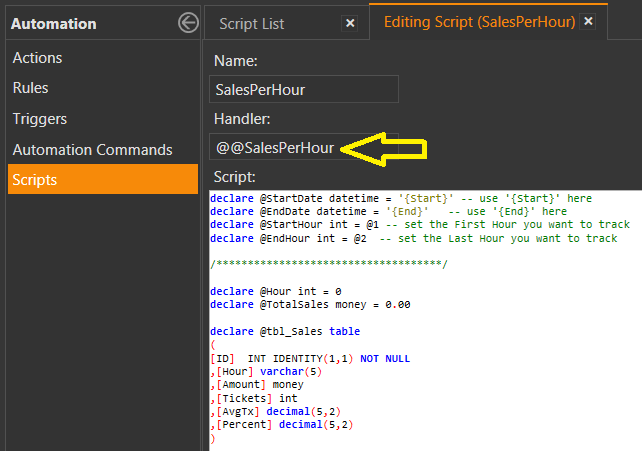Output: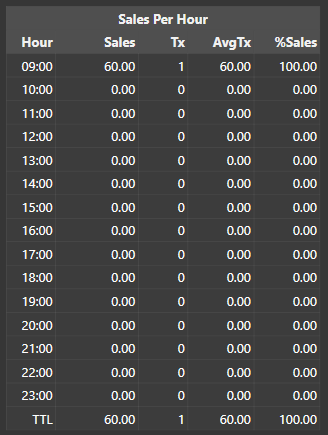3 Likes

@QMcKay

If you have a few minutes spare, could you kindly check my report/script as I cannot seem to get this working. I would be very grateful for any help. I’ve altered the times to 0 and 23.

Script:

``````declare @StartDate datetime = '{Start}'
declare @EndDate datetime = '{End}'
declare @StartHour int = @0 -- set the First Hour you want to track
declare @EndHour int = @23 -- set the Last Hour you want to track

/************************************/

declare @Hour int = 0
declare @TotalSales money = 0.00

declare @tbl_Sales table
(
[ID]  INT IDENTITY(1,1) NOT NULL
,[Hour] varchar(5)
,[Amount] decimal(9,2)
,[Tickets] int
,[AvgTx] decimal(9,2)
,[Percent] decimal(9,2)
)

set @Hour = @StartHour

WHILE @Hour < @EndHour+1
BEGIN
INSERT INTO @tbl_Sales
SELECT
@Hour as [Hour]
, SUM(CASE WHEN (DATEPART(hour,[Date])>=@Hour AND DATEPART(hour,[Date])<@Hour+1)
THEN [TotalAmount]
ELSE 0 END) as [Amount]
, COUNT(CASE WHEN (DATEPART(hour,[Date])>=@Hour AND DATEPART(hour,[Date])<@Hour+1)
THEN 1
ELSE null END) as [Tickets]
,0,0
FROM [dbo].[Tickets]
WHERE [Date]>=@StartDate AND [Date]<=@EndDate
SET @Hour = @Hour + 1
END

INSERT INTO @tbl_Sales
SELECT 'TTL', sum([Amount]), sum([Tickets]), 0, 0 FROM @tbl_Sales

set @TotalSales = (SELECT [Amount] FROM @tbl_Sales WHERE [Hour]='TTL')

UPDATE @tbl_Sales SET
[AvgTx] = (CASE WHEN [Tickets]=0 THEN 0 ELSE [Amount]/[Tickets] END)
,[Percent] = [Amount]/@TotalSales * 100

SELECT CASE
WHEN isnumeric([Hour])=1
THEN (CASE WHEN [Hour]<10 THEN '0'+[Hour]+':00' ELSE [Hour]+':00' END)
ELSE [Hour]
END as [Hour]
, [Amount], [Tickets], [AvgTx], [Percent]
FROM @tbl_Sales``````

Open it from actual reports section first before you try to preview.

You probably copy pasted his script right? So you probably didn’t read his instructions that said set your first and last hour. For the two declarations StartHour and EndHour

I copied and then set them to

`declare @StartHour int = @0 declare @EndHour int = @23`

Also changed my report to reflect the changed hours

Sorry i’m completely new to scripts so I don’t even know if that’s the correct way.

EDIT: Managed to figure it out, i deleted the “@” from
`declare @StartHour int = @0 declare @EndHour int = @23`

EDIT 2: Final resultLeave them 1 and 2 it tells it to read from the report. You don’t have to change anything in script.

So change it back to @1 and @2 and it will work fine. This way you only ever edit report in future if you need to change hours.

1 Like

Thanks a lot bro,this does really work for me.

Thanks a lot bro,this was great help for me.Wow I needed something like this, too!
By the way, is it possible to display the Profit Per Hour, alongside the gross sales?

I just get “Commandtext property has not been initialized.”

This is not as fancy as Q´s… but it does the trick! LOL…

``````[Tickets por Hora:1, 1, 1, 1]
>Time|Ticket Count|Total|Percent
{REPORT TICKET DETAILS:FT([T.Time],'hh'),T.TicketNumber.Count,[T.TotalAmount.Sum]-[CA.Discount.Sum]-[CA.Discount Fixed.Sum]-[CA.Taxa de Entrega.Sum],T.TotalAmount.Percent}
>Total:|{REPORT TICKET DETAILS:T.TicketNumber.Count,[T.TotalAmount.Sum]-[CA.Discount.Sum]-[CA.Discount Fixed.Sum]-[CA.Taxa de Entrega.Sum],T.TotalAmount.Percent}

[ORDERS:1, 1, 1, 1]
>Time|Order Count|Total|Percent
{REPORT ORDER DETAILS:FT([O.Time],'hh'),O.Id.Count,O.Total.Sum,O.Total.Percent}
>Total:|{REPORT ORDER DETAILS:O.Id.Count,O.Total.Sum,O.Total.Percent}
``````

Hope this helps

G.

3 Likes

Greatbut I don’t get it.

Is it this part that will “break” the report into individual hour?

What does FT mean? Format Text `Time` in hh ?

I undertsand that sum will group lines but I don’t understand how hour/Time (hh) becomes the group criteria.

Also I try:

To order by hours without success.

Marc

Hi, How to make it by Item Name? I need something like thatHey bro How to make u r Screen Shot Report I want to do like that can u show me how to do it
8-9 9-10 11-2 like that

``````Hi

Create a Report (Hourly Tickets Report):
``````

``````    [Hourly Tickets:3,1,1,1,1,1,1,1,1,1,1,1,1,1,1,1,1,1]
>Date/Hour|8-9|9-10|10-11|11-12|12-13|13-14|14-15|15-16|16-17|17-18|18-19|19-20|20-21|21-22|22-23|23-24|24-01
@@TicketCountByPeakHours
@@TicketAmount
>Total Tickets = @@TicketCountByPeakHoursTotals
>Total Amount = @@TicketAmountByPeakHoursTotal
``````

``````  **Script** @@TicketCountByPeakHours

SELECT Convert(nvarchar(20),Date,103),
Sum(CASE WHEN DATEPART(hour, [DATE]) BETWEEN 7 AND 8 THEN 1 ELSE 0 END),
Sum(CASE WHEN DATEPART(hour, [DATE]) BETWEEN 8.01 AND 9 THEN 1 ELSE 0 END),
Sum(CASE WHEN DATEPART(hour, [DATE]) BETWEEN 9.01 AND 10 THEN 1 ELSE 0 END),
Sum(CASE WHEN DATEPART(hour, [DATE]) BETWEEN 10.01 AND 11 THEN 1 ELSE 0 END),
Sum(CASE WHEN DATEPART(hour, [DATE]) BETWEEN 11.01 AND 12 THEN 1 ELSE 0 END),
Sum(CASE WHEN DATEPART(hour, [DATE]) BETWEEN 12.01 AND 13 THEN 1 ELSE 0 END),
Sum(CASE WHEN DATEPART(hour, [DATE]) BETWEEN 13.01 AND 14 THEN 1 ELSE 0 END),
Sum(CASE WHEN DATEPART(hour, [DATE]) BETWEEN 14.01 AND 15 THEN 1 ELSE 0 END),
Sum(CASE WHEN DATEPART(hour, [DATE]) BETWEEN 15.01 AND 16 THEN 1 ELSE 0 END),
Sum(CASE WHEN DATEPART(hour, [DATE]) BETWEEN 16.01 AND 17 THEN 1 ELSE 0 END),
Sum(CASE WHEN DATEPART(hour, [DATE]) BETWEEN 17.01 AND 18 THEN 1 ELSE 0 END),
Sum(CASE WHEN DATEPART(hour, [DATE]) BETWEEN 18.01 AND 19 THEN 1 ELSE 0 END),
Sum(CASE WHEN DATEPART(hour, [DATE]) BETWEEN 19.01 AND 20 THEN 1 ELSE 0 END),
Sum(CASE WHEN DATEPART(hour, [DATE]) BETWEEN 20.01 AND 21 THEN 1 ELSE 0 END),
Sum(CASE WHEN DATEPART(hour, [DATE]) BETWEEN 21.01 AND 22 THEN 1 ELSE 0 END),
Sum(CASE WHEN DATEPART(hour, [DATE]) BETWEEN 22.01 AND 23 THEN 1 ELSE 0 END),
Sum(CASE WHEN DATEPART(hour, [DATE]) BETWEEN 23.01 AND 24 THEN 1 ELSE 0 END)

FROM Tickets
Where Date > '{Start}' and Date < '{End}'
GROUP BY Convert(nvarchar(20),Date,103)
``````

``````**Script** @@TicketCountByPeakHoursTotals

SELECT Count(Id)
FROM Tickets
Where Date > '{Start}' and Date < '{End}'
``````

``````**Script** @@TicketAmount

SELECT 'Total of' + ' ' + min(convert(nvarchar,Date,105)),
Sum(CASE WHEN DATEPART(hour, [Date]) BETWEEN 7 AND 8 THEN TotalAmount ELSE 0 END),
Sum(CASE WHEN DATEPART(hour, [Date]) BETWEEN 8.01 AND 9 THEN TotalAmount ELSE 0 END),
Sum(CASE WHEN DATEPART(hour, [Date]) BETWEEN 9.01 AND 10 THEN TotalAmount ELSE 0 END),
Sum(CASE WHEN DATEPART(hour, [Date]) BETWEEN 10.01 AND 11 THEN TotalAmount ELSE 0 END),
Sum(CASE WHEN DATEPART(hour, [Date]) BETWEEN 11.01 AND 12 THEN TotalAmount ELSE 0 END),
Sum(CASE WHEN DATEPART(hour, [Date]) BETWEEN 12.01 AND 13 THEN TotalAmount ELSE 0 END),
Sum(CASE WHEN DATEPART(hour, [Date]) BETWEEN 13.01 AND 14 THEN TotalAmount ELSE 0 END),
Sum(CASE WHEN DATEPART(hour, [Date]) BETWEEN 14.01 AND 15 THEN TotalAmount ELSE 0 END),
Sum(CASE WHEN DATEPART(hour, [Date]) BETWEEN 15.01 AND 16 THEN TotalAmount ELSE 0 END),
Sum(CASE WHEN DATEPART(hour, [Date]) BETWEEN 16.01 AND 17 THEN TotalAmount ELSE 0 END),
Sum(CASE WHEN DATEPART(hour, [Date]) BETWEEN 17.01 AND 18 THEN TotalAmount ELSE 0 END),
Sum(CASE WHEN DATEPART(hour, [Date]) BETWEEN 18.01 AND 19 THEN TotalAmount ELSE 0 END),
Sum(CASE WHEN DATEPART(hour, [Date]) BETWEEN 19.01 AND 20 THEN TotalAmount ELSE 0 END),
Sum(CASE WHEN DATEPART(hour, [Date]) BETWEEN 20.01 AND 21 THEN TotalAmount ELSE 0 END),
Sum(CASE WHEN DATEPART(hour, [Date]) BETWEEN 21.01 AND 22 THEN TotalAmount ELSE 0 END),
Sum(CASE WHEN DATEPART(hour, [Date]) BETWEEN 22.01 AND 23 THEN TotalAmount ELSE 0 END),
Sum(CASE WHEN DATEPART(hour, [Date]) BETWEEN 23.01 AND 24 THEN TotalAmount ELSE 0 END)

FROM Tickets
Where Date > '{Start}' and Date < '{End}'
GROUP BY Convert(nvarchar(20),Date,105)
``````

``````**Script** @@TicketAmountByPeakHoursTotal

SELECT sum(TotalAmount)
FROM Tickets
Where Date > '{Start}' and Date < '{End}'``````
1 Like

i create report u said one it show this message
i will show script also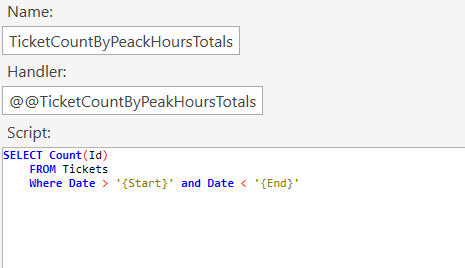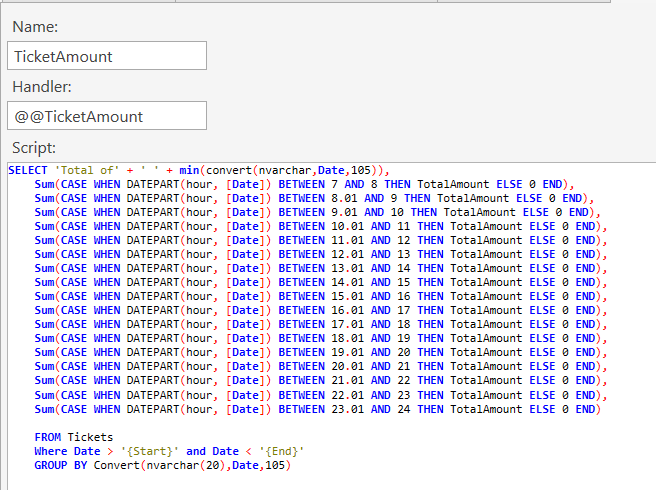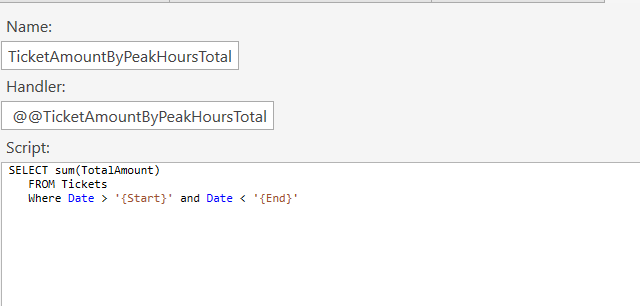i am doing right ?

Go to the reports module and view it there first then try the preview in report builder after that.